Test: Systems of Linear Equations, Matrix Algebra & Transform Theory- 2

# Test: Systems of Linear Equations, Matrix Algebra & Transform Theory- 2

Test Description

## 30 Questions MCQ Test GATE Mechanical (ME) 2023 Mock Test Series | Test: Systems of Linear Equations, Matrix Algebra & Transform Theory- 2

Test: Systems of Linear Equations, Matrix Algebra & Transform Theory- 2 for Civil Engineering (CE) 2023 is part of GATE Mechanical (ME) 2023 Mock Test Series preparation. The Test: Systems of Linear Equations, Matrix Algebra & Transform Theory- 2 questions and answers have been prepared according to the Civil Engineering (CE) exam syllabus.The Test: Systems of Linear Equations, Matrix Algebra & Transform Theory- 2 MCQs are made for Civil Engineering (CE) 2023 Exam. Find important definitions, questions, notes, meanings, examples, exercises, MCQs and online tests for Test: Systems of Linear Equations, Matrix Algebra & Transform Theory- 2 below.
Solutions of Test: Systems of Linear Equations, Matrix Algebra & Transform Theory- 2 questions in English are available as part of our GATE Mechanical (ME) 2023 Mock Test Series for Civil Engineering (CE) & Test: Systems of Linear Equations, Matrix Algebra & Transform Theory- 2 solutions in Hindi for GATE Mechanical (ME) 2023 Mock Test Series course. Download more important topics, notes, lectures and mock test series for Civil Engineering (CE) Exam by signing up for free. Attempt Test: Systems of Linear Equations, Matrix Algebra & Transform Theory- 2 | 30 questions in 90 minutes | Mock test for Civil Engineering (CE) preparation | Free important questions MCQ to study GATE Mechanical (ME) 2023 Mock Test Series for Civil Engineering (CE) Exam | Download free PDF with solutions
 1 Crore+ students have signed up on EduRev. Have you?
Test: Systems of Linear Equations, Matrix Algebra & Transform Theory- 2 - Question 1

### Consider the system of equations given below:  x + y =  2 2x + 2y = 5 This system has

Detailed Solution for Test: Systems of Linear Equations, Matrix Algebra & Transform Theory- 2 - Question 1

(b) This can be written as AX = B Where A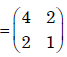Angemented matrix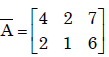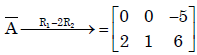rank(A) ≠ rank(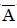). The system is inconsistant .So system has no solution.

Test: Systems of Linear Equations, Matrix Algebra & Transform Theory- 2 - Question 2

### For what value of a, if any, will the following system of equations in x, y and z have a solution?   2x + 3y = 4 x+y+z = 4 x + 2y - z = a

Detailed Solution for Test: Systems of Linear Equations, Matrix Algebra & Transform Theory- 2 - Question 2

(b)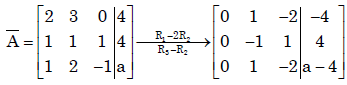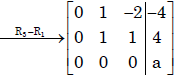If a = 0 then rank (A) = rank() = 2. Therefore the system is consistant
∴ The system has soln .

Test: Systems of Linear Equations, Matrix Algebra & Transform Theory- 2 - Question 3

### Solution for the system defined by the set of equations 4y + 3z = 8; 2x – z = 2 and 3x + 2y =5 is

Detailed Solution for Test: Systems of Linear Equations, Matrix Algebra & Transform Theory- 2 - Question 3

Ans.(d)
Consider the matrix A =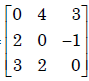,Now det (A) = 0
So byCramer's Rule the system has no solution

Test: Systems of Linear Equations, Matrix Algebra & Transform Theory- 2 - Question 4

For what values of α and β the following simultaneous equations have an infinite numberof solutions?
x + y + z = 5; x + 3y + 3z = 9; x + 2y + αz = β

Detailed Solution for Test: Systems of Linear Equations, Matrix Algebra & Transform Theory- 2 - Question 4

(d)=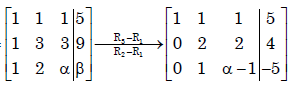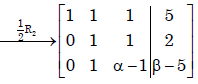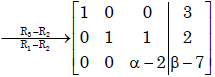For infinite solution of the system
α − 2 = 0 and β − 7 = 0
⇒ α = 2 and β = 7.

Test: Systems of Linear Equations, Matrix Algebra & Transform Theory- 2 - Question 5

Let A be a 3 × 3 matrix with rank 2. Then AX = 0 has

Detailed Solution for Test: Systems of Linear Equations, Matrix Algebra & Transform Theory- 2 - Question 5

(b)
We know , rank (A) + Solution space X(A) = no. of unknowns.
⇒2 + X(A) = 3 . [Solution space X(A)= No. of linearly independent vectors]
⇒ X(A) =1.

Test: Systems of Linear Equations, Matrix Algebra & Transform Theory- 2 - Question 6

A is a 3 x 4 real matrix and A x = b is an inconsistent system of equations. The highest possible rank of A is

Detailed Solution for Test: Systems of Linear Equations, Matrix Algebra & Transform Theory- 2 - Question 6

(b). Highest possible rank of A= 2 ,as Ax = b is an inconsistent system.

Test: Systems of Linear Equations, Matrix Algebra & Transform Theory- 2 - Question 7

Consider the matrices X (4 × 3), Y (4 × 3) and P (2 × 3). The order or P (XTY)–1PT] T will be

Detailed Solution for Test: Systems of Linear Equations, Matrix Algebra & Transform Theory- 2 - Question 7

(a)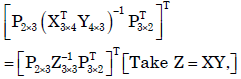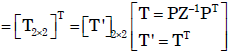Test: Systems of Linear Equations, Matrix Algebra & Transform Theory- 2 - Question 8

Given matrix [A] =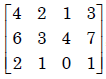the rank of the matrix is

Detailed Solution for Test: Systems of Linear Equations, Matrix Algebra & Transform Theory- 2 - Question 8

(c)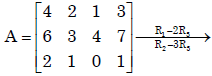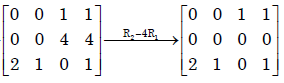∴Rank(A) = 2

Test: Systems of Linear Equations, Matrix Algebra & Transform Theory- 2 - Question 9

The Laplace transform of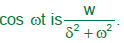Detailed Solution for Test: Systems of Linear Equations, Matrix Algebra & Transform Theory- 2 - Question 9

Ans. (b)False
Laplace transform of

Test: Systems of Linear Equations, Matrix Algebra & Transform Theory- 2 - Question 10

There are two containers, with one containing 4 Red and 3 Green balls and the other containing 3 Blue and 4 Green balls. One bal is drawn at random form each container.The probability that one of the ball is Red and the other is Blue will be

Test: Systems of Linear Equations, Matrix Algebra & Transform Theory- 2 - Question 11

If L defines the Laplace Transform of a function, L [sin (at)] will be equal to

Detailed Solution for Test: Systems of Linear Equations, Matrix Algebra & Transform Theory- 2 - Question 11

Ans. (b)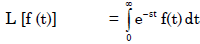⇒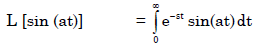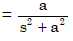Test: Systems of Linear Equations, Matrix Algebra & Transform Theory- 2 - Question 12

The Inverse Laplace transform of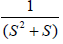is

Detailed Solution for Test: Systems of Linear Equations, Matrix Algebra & Transform Theory- 2 - Question 12

Ans. (c)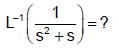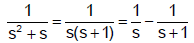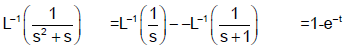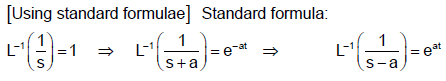Test: Systems of Linear Equations, Matrix Algebra & Transform Theory- 2 - Question 13

Laplace transform for the function f(x) = cosh (ax) is

Detailed Solution for Test: Systems of Linear Equations, Matrix Algebra & Transform Theory- 2 - Question 13

Ans. (b)
It is a standard result that
L (cosh at) =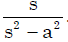Test: Systems of Linear Equations, Matrix Algebra & Transform Theory- 2 - Question 14

If F(s) is the Laplace transform of function f (t), then Laplace transform of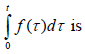Detailed Solution for Test: Systems of Linear Equations, Matrix Algebra & Transform Theory- 2 - Question 14

Ans. (a)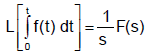Test: Systems of Linear Equations, Matrix Algebra & Transform Theory- 2 - Question 15

Laplace transform of the function sin ωt

Detailed Solution for Test: Systems of Linear Equations, Matrix Algebra & Transform Theory- 2 - Question 15

Ans. (b)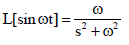Test: Systems of Linear Equations, Matrix Algebra & Transform Theory- 2 - Question 16

Laplace transform of (a + bt)2 where ‘a’ and ‘b’ are constants is given by:

Detailed Solution for Test: Systems of Linear Equations, Matrix Algebra & Transform Theory- 2 - Question 16

Ans.(c)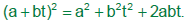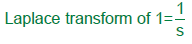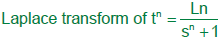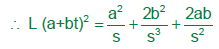Test: Systems of Linear Equations, Matrix Algebra & Transform Theory- 2 - Question 17

A delayed unit step function is defined as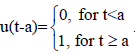Its Laplace transform is

Detailed Solution for Test: Systems of Linear Equations, Matrix Algebra & Transform Theory- 2 - Question 17

Ans. (d)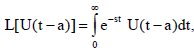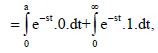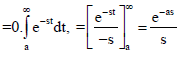Test: Systems of Linear Equations, Matrix Algebra & Transform Theory- 2 - Question 18

The Laplace transform of the function sin2 2t is

Detailed Solution for Test: Systems of Linear Equations, Matrix Algebra & Transform Theory- 2 - Question 18

Ans.(a)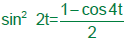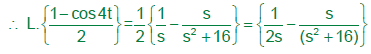Test: Systems of Linear Equations, Matrix Algebra & Transform Theory- 2 - Question 19

Find the rank of the matrix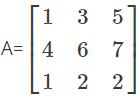Detailed Solution for Test: Systems of Linear Equations, Matrix Algebra & Transform Theory- 2 - Question 19

To find out the rank of the matrix first find the |A|
If the value of the |A| = 0 then the matrix is said to be reduced
But, as the determinant of A has some finite value, then the rank of the matrix is 3.

Test: Systems of Linear Equations, Matrix Algebra & Transform Theory- 2 - Question 20

The running integrator, given by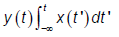Detailed Solution for Test: Systems of Linear Equations, Matrix Algebra & Transform Theory- 2 - Question 20

Ans. (b)

Test: Systems of Linear Equations, Matrix Algebra & Transform Theory- 2 - Question 21

The state transition matrix for the system  X- = AX with initial state X(0) is

Detailed Solution for Test: Systems of Linear Equations, Matrix Algebra & Transform Theory- 2 - Question 21

Ans. (c)

Test: Systems of Linear Equations, Matrix Algebra & Transform Theory- 2 - Question 22

The Fourier transform of x(t) = e–at u(–t), where u(t) is the unit step function

Detailed Solution for Test: Systems of Linear Equations, Matrix Algebra & Transform Theory- 2 - Question 22

Ans. (d)

Test: Systems of Linear Equations, Matrix Algebra & Transform Theory- 2 - Question 23

The fundamental period of the discrete-time signal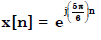is

Detailed Solution for Test: Systems of Linear Equations, Matrix Algebra & Transform Theory- 2 - Question 23

Ans. (b)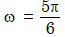or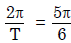or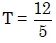Test: Systems of Linear Equations, Matrix Algebra & Transform Theory- 2 - Question 24

u(t) represents the unit step function. The Laplace transform of u(t – ζ) is

Detailed Solution for Test: Systems of Linear Equations, Matrix Algebra & Transform Theory- 2 - Question 24

Ans. (c)
f(t) = u(t – ζ)
L{f(t)} = L{u(t – ζ)}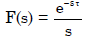Test: Systems of Linear Equations, Matrix Algebra & Transform Theory- 2 - Question 25

The fundamental period of x(t) = 2 sin πt + 3 sin 3πt, with t expressed in seconds, is

Detailed Solution for Test: Systems of Linear Equations, Matrix Algebra & Transform Theory- 2 - Question 25

Ans. (d)
H.C.F. of 2π and 3π is 6π.
Then, fundamental frequency = 6π
∴ Period, T =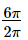= 3 sec

Test: Systems of Linear Equations, Matrix Algebra & Transform Theory- 2 - Question 26

If the Fourier transform of x[n] is X(e), then the Fourier transform of (–1)n x[n] is

Detailed Solution for Test: Systems of Linear Equations, Matrix Algebra & Transform Theory- 2 - Question 26

Ans. (c)

Test: Systems of Linear Equations, Matrix Algebra & Transform Theory- 2 - Question 27

Given f(t) and g(t) as shown below: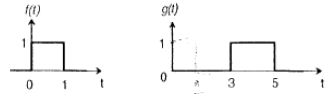g (t) can be expressed as

Test: Systems of Linear Equations, Matrix Algebra & Transform Theory- 2 - Question 28

Given f(t) and g(t) as shown below:The Laplace transform of g(t) is

Detailed Solution for Test: Systems of Linear Equations, Matrix Algebra & Transform Theory- 2 - Question 28

Ans. (c)

Test: Systems of Linear Equations, Matrix Algebra & Transform Theory- 2 - Question 29

The Laplace transform of g(t) is

Detailed Solution for Test: Systems of Linear Equations, Matrix Algebra & Transform Theory- 2 - Question 29

Ans. (c)

Test: Systems of Linear Equations, Matrix Algebra & Transform Theory- 2 - Question 30

Let Y(s) be the Laplace transformation of the function y (t), then final value of the function is

Detailed Solution for Test: Systems of Linear Equations, Matrix Algebra & Transform Theory- 2 - Question 30

Ans. (c)

## GATE Mechanical (ME) 2023 Mock Test Series

27 docs|243 tests
Information about Test: Systems of Linear Equations, Matrix Algebra & Transform Theory- 2 Page
In this test you can find the Exam questions for Test: Systems of Linear Equations, Matrix Algebra & Transform Theory- 2 solved & explained in the simplest way possible. Besides giving Questions and answers for Test: Systems of Linear Equations, Matrix Algebra & Transform Theory- 2, EduRev gives you an ample number of Online tests for practice

## GATE Mechanical (ME) 2023 Mock Test Series

27 docs|243 tests(Scan QR code)